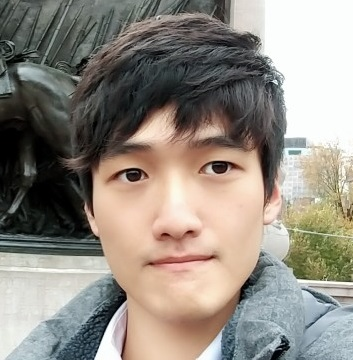Jaewoo Song

### Categories

• Tech

Since the basic idea seeing the overall input contexts as references is same, it is obvious that we can use attention to basic RNN based models.

In other words, there are various application methods so I cannot say that the examples below are absolutely only correct options.

Therefore, we should understand that the basic idea is taken equally but the specific way can vary depending on users, purposes or tasks.

Seeing previous hidden states to produce an output

To make a token produced from one RNN cell, we can check the similarity with current hidden state and previous hidden states which the model went through.

That is, we make a token based on the context of sequence before.Basically, it is same as the usage in the decoder of seq2seq model.

But there is no encoder which contains full context or all hidden states, so we just focus on previous hidden states which the model already passed.

The rest of the process, which includes softmax and weighted sum to make attention value, is no different.

This can be used for text generation with basic RNN model.

Seeing overall output from RNN cells after finishing the whole process

We can also use attention technique after getting all outputs(hidden states) from RNN.

This idea implies that the importance score of each output can be interpreted as attention weight and can be applied to original output values.

Here’s a simple example for this.Assume that the sequence length is $L$ and the size of a hidden state is $h$.

We have the output tensor in shape of $(L, h)$. (We are not considering batch size right now.)

This output tensor goes through a linear layer in size of $h \times 1$.

So this output vectors are converted into scalar values, which are attention scores.

Then after a softmax, these values become attention weights.

And original output tensor is put into weighted sum process based on this attention weights, which leads to attention value in size of $h$.

This attention value(vector) is concatenated with original output vector then put into additional procedure based on tasks the user wants to conduct.

I used this method in text classification by putting additional linear layer and softmax after the concatenation for final classification.

In this case, the linear layer which converts output hidden states into vectors in size $1$ becomes $Q$(Query) itself.

Since it is just a linear transformation, so this linear transformation matrix is Query which asks how each hidden state can be changed into a value showing us its importance.

The original hidden states are $K$(Key) and final weighted sum(in the same size with $h$) becomes $V$(Value).

As I mentioned, the attention’s idea can be adapted into various circumstances as various forms.

The point is not the examples above, but the fundamental idea itself.

So that’s all I need to say about the attention mechanism.

Now we are ready to see the famous Transformer model.